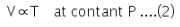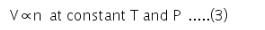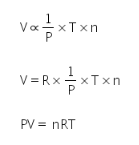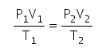# Derive ideal gas equation from diff.laws

Derive ideal gas equation from diff.laws

The gas equation is an equation used in chemical equations for calculating the changes in the volume of gases when pressure and temperature both undergo a change, thereby giving a simultaneous effect of changes of temperature and pressure on the volume of a given mass of dry gas.

According to Boyle’s law: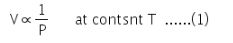According to Charles’ law: